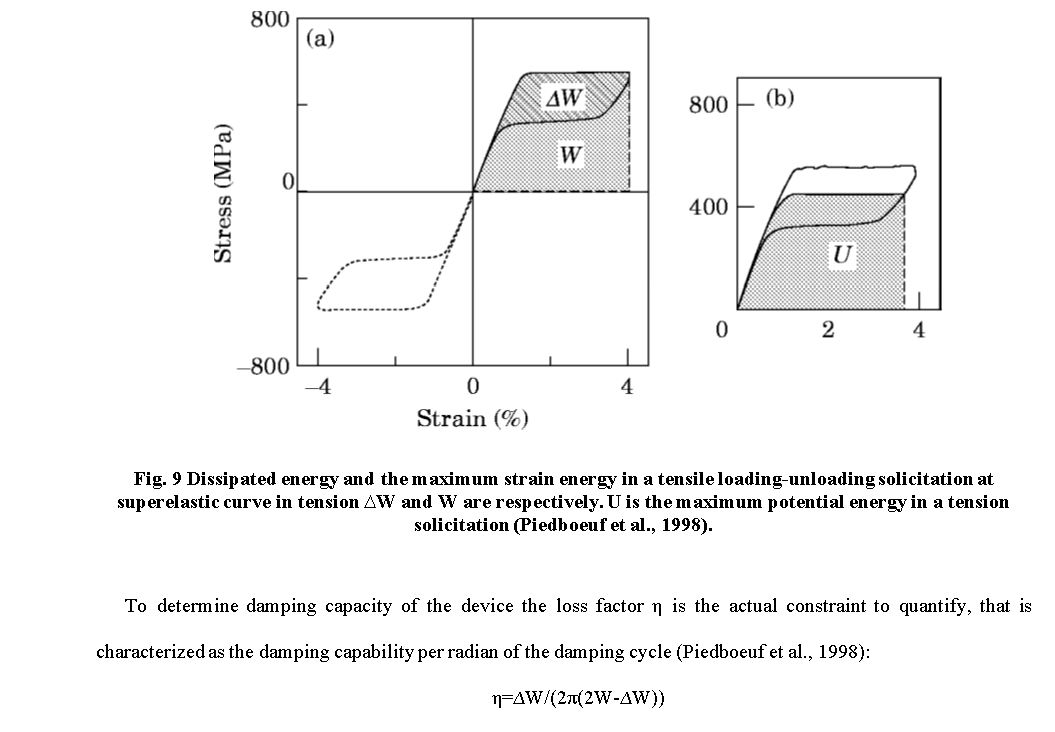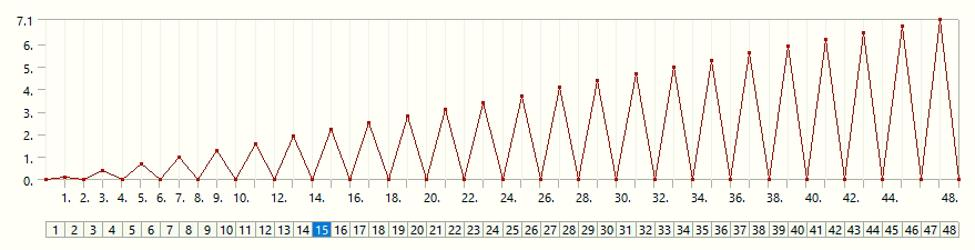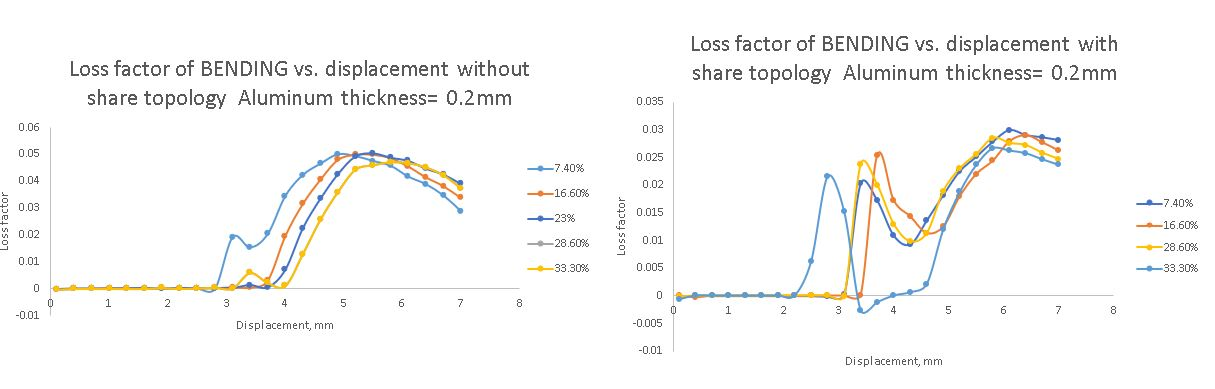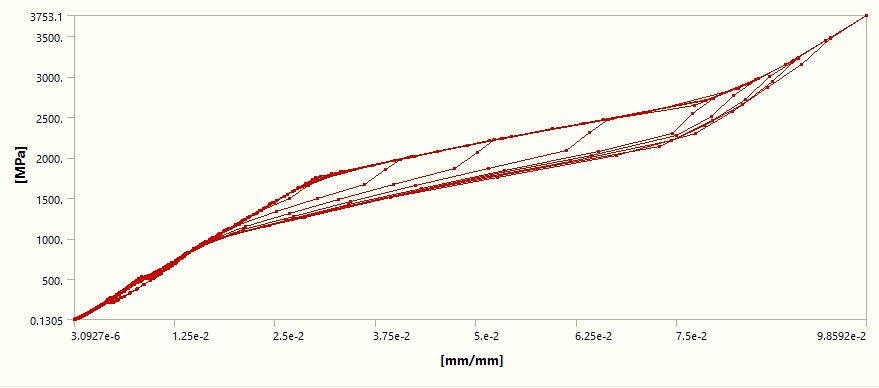## General Mechanical

•Nurs134
Subscriber

Good morning,

I am working on a sandwich plate of bonded two materials Nitinol+aluminum+Nitinol. I have set up a bonded contact between the materials. I have set fixed support to the plate from both sides. A downward displacement acting on the middle of the plate. Below are the two error messages and the warning message I receive when trying to solve it. Any help would be greatly appreciated, thank you.

Face split on the side of a sandwich plate where is necessary to take results.

An internal solution magnitude limit was exceeded. Please check your Environment for inappropriate load values or insufficient supports.  You may select the offending object and/or geometry via RMB on this warning in the Message window.  Please see the Troubleshooting section of the Help System for more information.

Displacement begins from 0.1mm to 3.7mm gradually with increment 0.3mm

Mesh was refined at the region of bending

Large deflections ON

Solver pivot warnings or errors have been encountered during the solution.  This is usually a result of an ill conditioned matrix possibly due to unreasonable material properties, and under constrained model, or contact related issues.  Check results carefully.

•peteroznewman
Subscriber

I recommend you use DesignModeler or SpaceClaim to create geometry with Shared Topology used so you can delete the Bonded Contact.

•Nurs134
Subscriber

Thanks a lot for the tip. The model was designed in the design modeler. Problem was avoided by changing mesh method from Multizone to face or body meshing

•Nurs134
Subscriber

My research is to determine the effect of changing the thickness of the damping material. At the end of static analysis, I need the result of the loss factor comparison for each mass to weight ratio of damping material in a sandwich structure.

I did the bending of the sandwich plate to calculate the loss factor from the hysteresis loop, by this formula:At each cycle of bending, equivalent max stress vs total equivalent total strain shows hysteresis loop, where I calculate the loss factor. Results were obtained for the sandwich plate with share topology and without it.The question: Is it right to use share topology in my case? Because the graph with share topology shows unphysical behavior. Or I should not use share topology?

•peteroznewman
Subscriber

I don't understand what you are plotting in the last two graphs.

Damping is a function of velocity and is seen in a dynamic analysis where there is velocity and is not present in a static analysis.

There are other mechanisms of dissipation that don't depend on velocity, such as plasticity, but I don't think you are using that in your model.

•Nurs134
Subscriber

Also, it is possible to calculate the loss factor by the ratio of area under the stress-strain curve in the hysteresis loop according to the formula above. Archive of bending attached:•peteroznewman
Subscriber
•peteroznewman
Subscriber

I am not expert at Shape Memory materials, but the ANSYS Help has a reference that you might find useful.

Auricchio, F., D. Fugazza, R. DesRoches. “Numerical and Experimental Evaluation of the Damping Properties of Shape-Memory Alloys.” Journal of Engineering Materials and Technology. 128: 3 (2006): 312-319.

•peteroznewman
Subscriber
•Nurs134
Subscriber

How to recreate such behavior in Ansys performing bending loads? And where are you plotted the graph?

•peteroznewman
Subscriber

This is a material property. It doesn't matter if the stress was caused by bending or tension.

I used the Nitinol material you defined in the attached model on a cube of material, meshed that cube with one linear element, used three planes of symmetry then applied a displacement on one face to stretch it out and then back to zero in a two-step analysis.

Draw a 10mm x 10mm x 10mm cube, use Displacement on one face of 1 mm in Step 1 and set it to 0 in Step 2. That is a strain of 0.1 In the two step analysis, make each step have 100 substeps and turn off Auto Time Stepping. Solve.

Copy the Stress Tabular Results into Excel.
The time column will go from 0 to 1 to 2 over 200 rows.
Create a new column called Strain.
For rows 1-100, the strain formula is Time*0.1
For rows 101-200, the strain formula is (2-Time)*0.1

Now plot Stress vs Strain.

However, you don't even need ANSYS to compute this. The graph matches the data in the material input table. Just use E and the four values of stress and you can create this plot by hand.

•Nurs134
Subscriber

Thanks, I get it. But why in ANSYS stress-strain curve was linear? For example in my case max eq.stress vs max eq.strain=linear, but min eq.stress vs max eq.strain=hysteresis do you know the explanation for such representation? Maybe it is due to aluminum involved in the structure?

If yes, how I can find the loss of energy for aluminum and for nitinol? Should I choose bode by body and represent their stress -strain curve separately?

•peteroznewman
Subscriber

I don't know why the plot of Strain as computed by ANSYS has all those kinks in it when the Strain as computed by hand is linear. Someone from ANSYS will have to answer that question.  In that example, there was only one material, the Nitinol.

How will you introduce an energy loss for the aluminum, since there is no hysteresis in the stress-strain curve of the material model for aluminum?  You can add damping to that material that will be applied in a dynamic analysis.